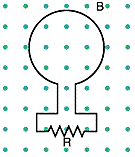# Problem: The magnetic flux through the loop shown in the figure below increases according to the relation ΦB =6.0t2 + 6.4t, where ΦB is in milliwebers and t is in seconds.(a) What is the magnitude of the emf induced in the loop when t = 2.9 s?mV(b) What is the direction of the current through R?leftright    insufficient information

###### FREE Expert Solution

In this problem, we'll apply Faraday's law to determine the magnitude of the induced emf and Lenz's law to determine the direction.

Induced emf:

$\overline{){\mathbf{\epsilon }}{\mathbf{=}}{\mathbf{N}}\frac{\mathbf{∆}\mathbf{\varphi }}{\mathbf{∆}\mathbf{t}}}$, where Φ  is the magnetic flux, N is the number of turns, t is the time, and A is the cross-sectional area of the coil.

Lenz's law: The induced current flows in a direction such that it opposes the change in flux inducing it.

Power rule of derivation:

$\overline{)\frac{\mathbit{d}}{\mathbit{d}\mathbit{t}}\mathbf{\left(}{\mathbit{x}}^{\mathbit{n}}\mathbf{\right)}{\mathbf{=}}{\mathbit{n}}{{\mathbit{x}}}^{\mathbit{n}\mathbf{-}\mathbf{1}}}$

97% (118 ratings)###### Problem Details

The magnetic flux through the loop shown in the figure below increases according to the relation ΦB =6.0t2 + 6.4t, where ΦB is in milliwebers and t is in seconds.(a) What is the magnitude of the emf induced in the loop when t = 2.9 s?

mV

(b) What is the direction of the current through R?

left

right

insufficient information